# 基于PGSA的S公司配送中心选址研究The Research on the Location of S Company’s Distribution Center Based on PGSA

• 全文下载: PDF(1720KB)    PP.17-31   DOI: 10.12677/SEA.2019.82003
• 下载量: 30  浏览量: 76

S公司是中国知名在线办公自动化解决方案提供商，主营纸张、耗材、文具、设备、日常用品等数万种办公用品，2016年《财富》世界500强企业。S公司凭借先进的供应链管理和全球采购体系，为用户提供一站式的行政办公采购服务。但随着S公司华东地区业务范围的不断扩张及业务量的快速增长，现有的松江仓已无法满足S公司日益增长的需求，建立新的配送中心迫在眉睫。在此背景下，本文通过分析S公司过往销售数据，以客户所在城市为标准，筛选出13个目标服务城市，利用模拟植物生长算法确定S公司配送中心选址坐标最优点及次优点，并结合坐标当地现实因素分析所得结果的可行性，为S公司配送中心选址提供具有现实意义的参考。首先，本文在分析S公司配送中心选址问题的基础上构建了相应的多目标选址模型。第二，为求解所建模型，在分析S公司配送中心选址问题影响因素的基础上，根据评价指标选取原则，建立了S公司配送中心选址指标体系，并详细介绍了指标权重的计算方法。第三，本文改进了模拟植物生长算法并对改进后算法有效性进行了验证，利用改进后算法求解所建模型，通过MATLAB进行计算得出S公司配送中心的选址地点。

S Company is a well-known online office automation solution provider in China. It specializes in tens of thousands of office supplies such as paper, consumable, stationery, equipment and daily necessities. It is the Fortune Global 500 in 2016. S Company with advanced supply chain man-agement and global procurement system is to provide users with one-stop administrative office procurement services. However, with the continuous expansion of the business scope of S Company in East China and the rapid growth of business volume, the existing Songjiang Warehouse has been unable to meet the growing demand of S Company and establishing a new distribution center is imminent. In this context, this paper analyzes the historical sales data of S Company, uses the city where the customer is as the standard, filters out 13 target service cities, and uses the PGSA to determine the best and the second advantage of S Company’s distribution center’s location coordinates. The feasibility of the results obtained from the analysis of the local realistic factors of the coordinates provides a realistic reference for the S Company’s distribution center site selection. Firstly, this paper builds a multi-objective location model on the basis of analyzing the S company’s distribution problem. Secondly, in order to solve the established model, based on the analysis of the influencing factors of the distribution center’s location problem of S company, according to the principle of evaluation index selection, the establishment of the S company’s distribution center location index system was established, and the calculation method of index weights was introduced in detail. Thirdly, the paper improves the simulation plant growth algorithm and verifies the effectiveness of the improved algorithm. The improved algorithm was used to solve the model, and the location of S company’s distribution center was calculated by MATLAB. Finally, according to the results of this study, the outlook and deficiencies are proposed.

1. 研究内容与研究方法

1.1. 研究内容

1) 学习配送中心相关基础概念，总结选址问题发展历程与经典模型。

2) 在分析S公司配送中心选址问题的基础上引出其本质——多设施韦伯问题。

3) 构建S公司配送中心选址问题的模型，详细介绍选址原则、模型的建立、评价指标体系的建立及其权重的确定以及华东地区各目标坐标点的转换。

4) 分析现有求解方法的局限性和模拟植物生长算法解决本文问题的适用性，根据模拟植物生长算法不足之处提出改进方法，并通过算例进行改进后算法有效性的验证。

1.2. 研究方法

1.3. 论文创新点

1) 本文在建立S公司配送中心选址模型时考虑了各配送中心之间的“排斥作用”，建立的模型是一个包含总权重距离最小和各配送中心间距离最大的多目标规划模型，区别于传统的多设施连续选址模型，使模型更具有实际应用意义。

2) 本文针对模拟植物生长算法新枝生长方向单一的问题，将原有算法中的固定生长角度改进为可变角度，从而提高算法收敛速度和寻优能力。

2. S公司配送中心选址模型建立

2.1. S公司配送中心选址问题描述

S公司是由世界500强、全球办公服务行业领袖企业——美国STAPLES公司在中国投资成立的外商独资企业。美国STAPLES公司是全球卓越的办公用品公司，可根据客户的需求来提供一站式办公用品采购服务，公司成立于1986年，总部位于美国马萨诸塞州的波士顿。目前S公司在全球拥有2100余家办公用品超市和仓储分销中心，全球员工总数超79000名，业务涉及20多个国家和地区。2004年，S公司来到中国在始终坚持其经营理念“Make It Easy”的基础上结合我国市场特点丰富了其服务内容。公司主营商用设备、电子数码、日用百货、办公文仪、家居礼品共五大类数万种办公用品。S公司的经营模式分为自营与外包两类。其中自营地区所涉及的商品配送服务由S公司自有物流或是供应商提供。S公司在华东地区现有的配送中心位于上海市松江区，主要负责上海、浙江和江苏三个地区的商品配送服务。随着S公司近年来一直积极推进“互联网+”政企采购平台电商化进程，公司业务范围不断扩大，与诸如中国移动、中国联通、国家电网等大客户达成长期合作计划，松江仓目前已远远无法满足S公司现有业务量：2017年11月单月销售额创历史新高，较原有记录增长143%；2017年12月8日单日销售额创历史新高，较原有记录增长159%；2017年全年销售额同比增长超55%。业务量的急剧增加导致松江仓屡次出现爆仓现象，严重影响了公司口碑及客户满意度。因此，针对当前国内特别是华东地区高速发展的业务需求，相对短缺的供给状况，公司决策层针计划在江浙沪地区建立新的配送中心，以提升物流效率，更好的实现公司“三天必达”的服务承诺，挖掘市场潜力，抢占市场份额。

2.2. 模型的建立

① 每个目标点客户需求一定，不随时间大幅波动；

② 所有运输路径上运输速度恒定，为同一常数；

③ 各配送中心到各目标服务点的距离可近似为直线距离；

④ 各配送中心不存在同时断货情况；

⑤ 各配送中心有足够的配送能力，可一次性配送完成客户所需物品；

⑥ 各配送中心均以相同概率为所有客户提供配送服务。

$\begin{array}{l}\mathrm{min}U=\underset{j=1}{\overset{m}{\sum }}\underset{i=1}{\overset{n}{\sum }}{w}_{ij}‖{A}_{i}-{P}_{j}‖\\ \mathrm{max}{D}_{j}=‖{P}_{1}-{P}_{j}‖\\ \mathrm{max}{D}_{jk}=‖{P}_{j}-{P}_{k}‖\\ s.t\left\{\begin{array}{l}{A}_{i},{P}_{j}\in {R}^{2},{w}_{ij}\ge 0\\ i=1,2,\cdots ,n\\ j=1,2,\cdots ,m\\ k=2,3,\cdots ,m\\ j>k\end{array}\right\}\end{array}$ (1)

2.3. 算法选择

2.3.1. 现有方法的局限性

2.3.2. 模拟植物生长算法对该问题的适用性

2.4. 坐标转换

WGS-84坐标是一种地固坐标系：采用WGS-84椭球，坐标原点为地球质心，X轴指向BIH1984.0零度子午面和CTP赤道的交点，Z轴指向BIH1984.0定义的协议地球极CTP方向，Y轴和Z、X轴构成右手坐标系  。

$\left\{\begin{array}{l}x=X+\frac{N}{2}t{\mathrm{cos}}^{2}B{l}^{2}+\frac{N}{24}t{\mathrm{cos}}^{4}B\left(5-{t}^{2}+9{\eta }^{2}+4{\eta }^{4}\right){l}^{4}\\ \text{\hspace{0.17em}}\text{\hspace{0.17em}}\text{\hspace{0.17em}}+\frac{N}{720}t{\mathrm{cos}}^{6}B\left(61-58{t}^{2}+{t}^{4}+270{\eta }^{2}-330{\eta }^{2}{t}^{2}\right){l}^{6}+\cdots \\ y=N\mathrm{cos}Bl+\frac{N}{6}{\mathrm{cos}}^{3}B\left(1-{t}^{2}+{\eta }^{2}\right){l}^{3}+\frac{N}{120}{\mathrm{cos}}^{5}B\left(5-18{t}^{2}+{t}^{4}+14{\eta }^{2}-58{\eta }^{2}{l}^{2}\right){l}^{5}+\cdots \end{array}\right\}$ (2)

$\left\{\begin{array}{l}X={A}_{0}\frac{B}{\rho }-{B}_{0}\mathrm{sin}B\mathrm{cos}B-{C}_{0}{\mathrm{sin}}^{3}B\mathrm{cos}B-{D}_{0}{\mathrm{sin}}^{5}B\mathrm{cos}B-{E}_{0}{\left(\mathrm{sin}\right)}^{7}\mathrm{cos}B\\ {e}^{2}=\frac{{a}^{2}-{b}^{2}}{{a}^{2}}\\ d=\frac{{b}^{2}}{a}=a\left(1-{e}^{2}\right)\\ {A}_{0}=d\left(1+\frac{3}{4}{e}^{2}+\frac{45}{64}{e}^{4}+\frac{175}{256}{e}^{6}+\frac{11025}{16384}{e}^{8}+\cdots \right)\\ {B}_{0}=d\left(\frac{3}{4}{e}^{2}+\frac{45}{64}{e}^{4}+\frac{175}{256}{e}^{6}+\frac{11025}{16384}{e}^{8}+\cdots \right)\\ {C}_{0}=d\left(\frac{15}{32}{e}^{4}+\frac{175}{368}{e}^{6}+\frac{3675}{8192}{e}^{8}+\cdots \right)\\ {D}_{0}=d\left(\frac{35}{96}{e}^{6}+\frac{735}{2048}{e}^{8}+\cdots \right)\\ {E}_{0}=d\left(\frac{315}{1024}{e}^{8}+\cdots \right)\end{array}\right\}$ (3)

X代表从赤道到B维度的子午线弧长；

${\eta }^{2}={e}^{2}{\mathrm{cos}}^{2}B$ 为辅助变量；

$N=\frac{{a}^{2}}{b\sqrt{1+{\eta }^{2}}}$ 为卯酉圈曲率半径；

${e}^{2}=\frac{{a}^{2}-{b}^{2}}{{a}^{2}}$ 为第二偏心率平方；

$t=\mathrm{tan}B$ 为辅助变量；

$l=L-{L}_{0}$ 为椭球点经度与相应中央子午线的差值；

a，b分别为参考椭球的长短半径。

3. 模拟植物生长

3.1. 模拟植物的生长演绎方式

L-系统遵循局部决定系统的生长法则，每个单元的行为只与它周围的单元行为有关，每个单元的无意识行为造成了整体系统的涌现。对植物生长做形式化的描述，可以根据以下几点进行  ：1) 破土而出的茎杆在一些叫做节的部位长出新枝；2) 大多数新枝上又长出更新的枝，这种分枝行为反复进行；3) 不同的枝彼此有相似性，整个植物有自相似结构。

1：a → c[b]d；2：b → a； 3：c → c；4：d → c(e)a；5：e → d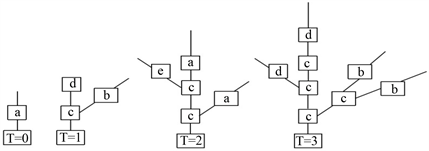Figure 1. Growth of a branch in an OL-systemTable 1. Branch growth process

3.2. 模拟植物生长算法

$P\text{\hspace{0.17em}}Mt=\frac{f\left(S0\right)-f\left(SMi\right)}{\underset{i=1}{\overset{t}{\sum }}\left[f\left(S0\right)-f\left(SMi\right)+\underset{j=1}{\overset{k}{\sum }}f\left(S0\right)-f\left(SMj\right)\right]}$ (4)

$Pmj=\frac{f\left(S0\right)-f\left(SMj\right)}{\underset{i=1}{\overset{t}{\sum }}\left[f\left(S0\right)-f\left(SMi\right)+\underset{j=1}{\overset{k}{\sum }}f\left(S0\right)-f\left(SMj\right)\right]}$ (5)

$\underset{i=1}{\overset{t}{\sum }}\underset{j=1}{\overset{k}{\sum }}\left(PMi+Pmi\right)=1$ (6)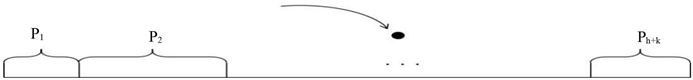Figure 2. State space of Morphin concentration

4. 模拟植物生长算法的改进

4.1. 初始生长点的确定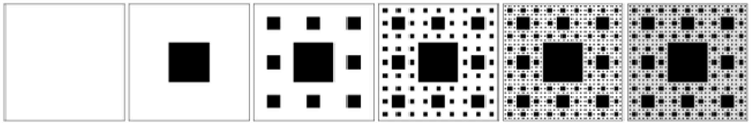Figure 3. Shelbins base blanket

4.2. 新枝生长方向的确定

4.3. 改进后算法有效性验证

$\begin{array}{l}\mathrm{min}f\left(x\right)={x}_{1}^{2}+{x}_{2}^{2}\\ s.t.-30<{x}_{1},{x}_{2}<30\end{array}$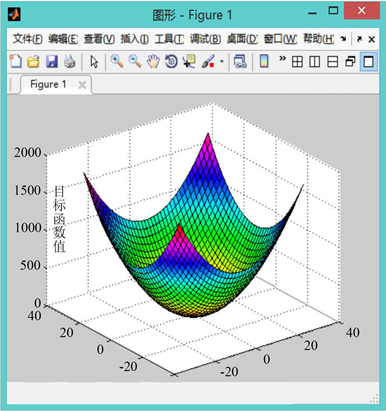Figure 4. Function feasible region

1) 比较图5图9的A曲线(改进前的模拟植物生长算法)可以发现原算法对初始生长点的选取有较大的依赖性，若初始生长点的选取靠近最优解，原算法可以较快的找到最优解，否则则会影响算法的求解速度，不利于大规模的计算。

2) 相比于原算法，引入有限元思想，利用谢尔宾斯基地毯直接设定生长点的改进策略使算法收敛速度的稳定性得到了保障，有效避免了由于初始生长点选取不当而造成的算法收敛过慢的现象(B曲线迭代次数均在600次左右)，且总能取到最优解，不会产生收敛于局部最优解的现象。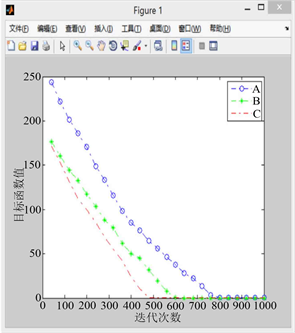Figure 5. When the random growth point of curve A is (16.5, 14.5)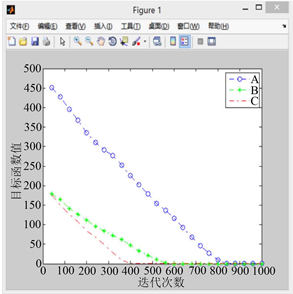Figure 6. When the random growth point of curve A is (14.2, 9.0)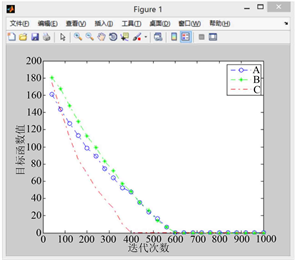Figure 7. When the random growth point of curve A is (−9.0, −10.1)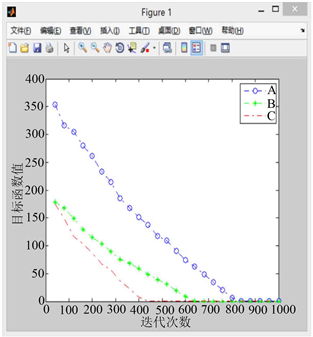Figure 8.When the random growth point of curve A is (9.2, −17.6)图8. 当A曲线随机生长点为(9.2, −17.6)时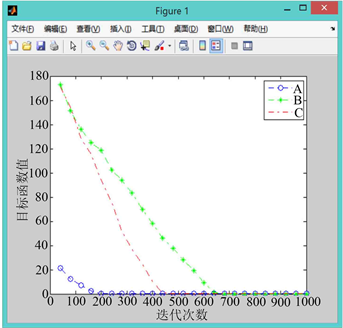Figure 9. When the random growth point of curve A is (4.3, −3.3)

3) 对比B曲线与C曲线可以看出，同时采用直接设定生长点与采用随机生长角度的改进后算法与原算法相比，收敛速度有了进一步的提升，算法计算效率比仅采用直接设定生长点进行改进的算法提高了30%左右，在保证寻优能力与收敛速度稳定性的基础上增加了运算效率。

4.4. 改进后PGSA的算法步骤与流程

Step 1：确定h个初始生长点 ${a}_{j}\in X$ ，X为目标函数的可行域，初始生长点为谢尔宾斯基地毯中正方形的顶点；

Step 2：求解各生长点形态素浓度(生长概率)：

${p}_{j}=\frac{1}{2}\left[\frac{\underset{i=1}{\overset{n}{\sum }}\frac{1}{w|{\alpha }_{j}{A}_{i}|}}{\underset{j=1}{\overset{h}{\sum }}\underset{i=1}{\overset{n}{\sum }}\frac{1}{w|{\alpha }_{j}{A}_{i}|}}+\frac{\underset{k=1}{\overset{m-1}{\sum }}|{a}_{j}{P}_{k}|+\underset{k=1}{\overset{m-1}{\sum }}\underset{r=2}{\overset{m-1}{\sum }}|{P}_{k}{P}_{r}|}{\underset{j=1}{\overset{h}{\sum }}\left(\underset{k=1}{\overset{m-1}{\sum }}|{a}_{j}{P}_{k}|+\underset{k=1}{\overset{m-1}{\sum }}\underset{r=2}{\overset{m-1}{\sum }}|{P}_{k}{P}_{r}|\right)}\right]$ (4-4)

Step 3：以Step 2中所得各点的生长概率为标准，构建各个生长点在0~1范围内的概率空间，用随机数来选择本次要迭代的生长点 ${a}_{j}$

Step 4：确定树枝长度(通常为L/1000，L代表目标函数可行域的长度)，生长点 ${a}_{j}$ 长出新枝后得到新的生长点，通过式4-4得出新生长点的生长概率，选出其中的最优点来替换 ${a}_{j}$

Step 5：若无新生长点产生如且完成了设定的迭代次数，则停止计算，输出局部最优解和全局最优解，不然转回Step 2。

5. 案例计算

5.1. 研究区域的选取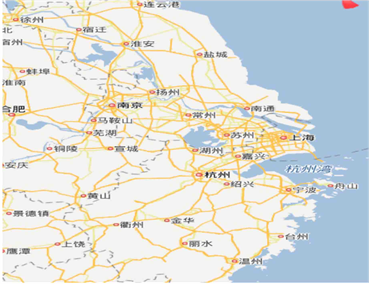Figure 10. Administrative area map of Jiangsu, Zhejiang and Shanghai

5.2. 坐标转换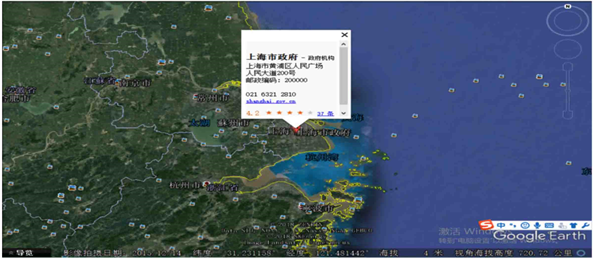Figure 11. Acquisition of coordinates of each target city in Google Earth (taking Shanghai as an example)Table 2. Coordinates and weights of each target city

5.3. 模型计算及分析Table 3. Partial local optimal solutions of the second site selectionTable 4. Partial local optimal solutions of the third site selection

6. 总结

1) 本文在分析研究问题后引出了S公司配送中心选址的韦伯型多设施问题，并根据实际运用考虑，在选址模型中加入了配送中心之间的“排斥”作用，区别于传统的韦伯型连续选址模型。

2) 本文为求解构建的S公司配送中心选址模型，建立了相应的评价指标体系，且为了保证赋权的公正客观，使用了一种组合赋权法；并完成华东地区各目标坐标点的坐标转换，将选址问题与GIS学相结合。

3) 根据模拟植物生长算法存在的不足之处提出改进方案：利用有限元思想直接设定初始生长点与改变新枝生长角度，并通过算例验证了改进后算法的有效性。

NOTES

*第一作者。

  李彤, 王春峰, 等. 求解整数规划的一种仿生类全局优化算法——模拟植物生长算法[J]. 系统工程理论与实践, 2005, 25(1): 76-85.  谢飞, 郭正鑫. 集中常用坐标转换方法的比较分析[J]. 现代测绘, 2017, 40(2): 53-55.  韦艳, 陈华根. GPS定位显示中的坐标转换[J]. 海洋测绘, 2010, 30(3): 16-19.  戴更新, 于龙振, 陈常菊. 基于混合遗传算法的多配送中心选址问题研究[J]. 物流技术, 2006(6): 38-42.  李彤, 王众托. 模拟植物生长算法在设施选址问题中的应用[J]. 系统工程理论实践, 2008, 28(12): 107-115.  Peter, K., Oliver, W., Sorkine-Hornung, A., Sorkine-Hornung, O., Smolic, A. and Gross, M. (2013) Finite Element Image Warping. Computer Graphics Forum, 32, 31-39. https://doi.org/10.1111/cgf.12023  李彤. 基于模拟植物生长的二级整数规划算法研究[D]: [博士学位论文]. 天津: 天津大学, 2005.